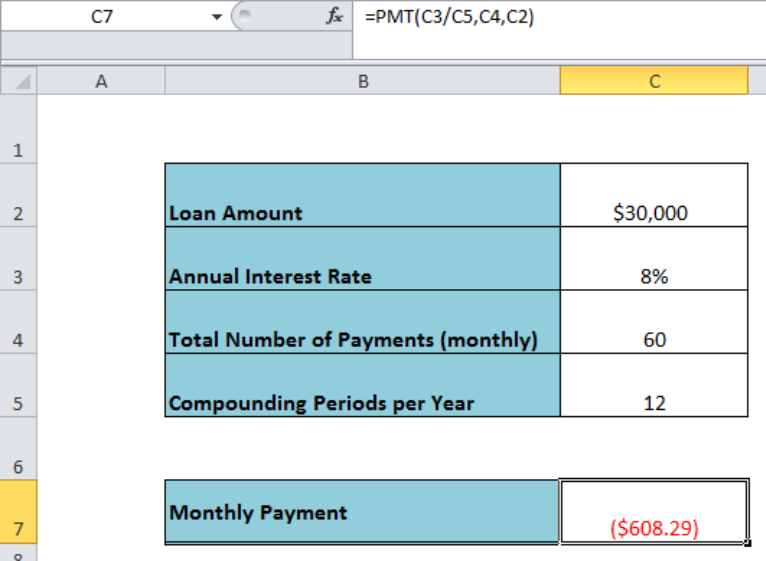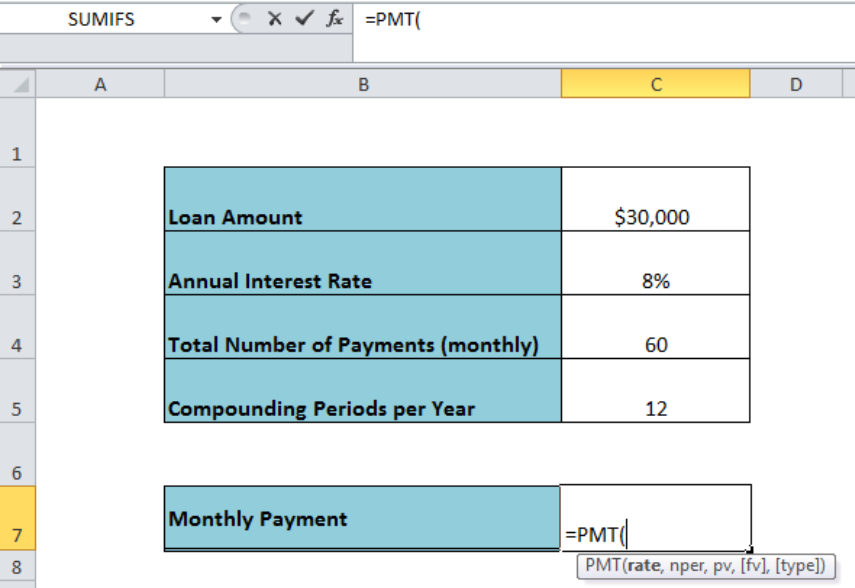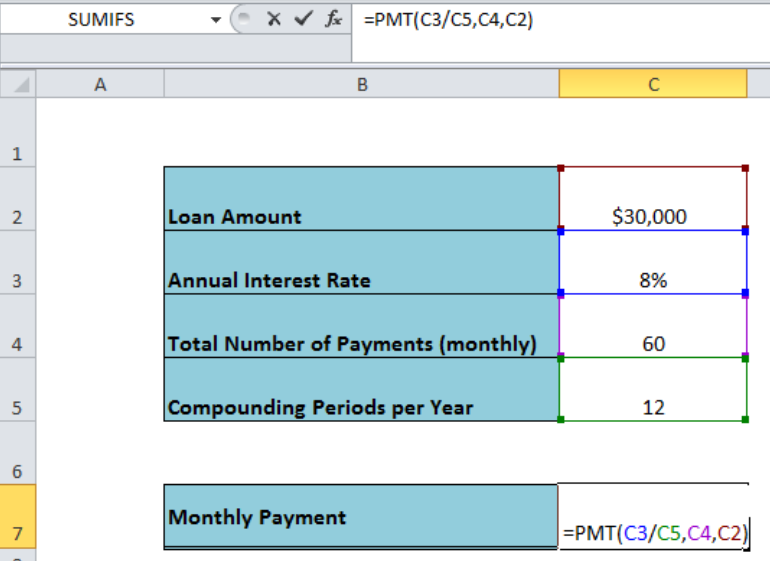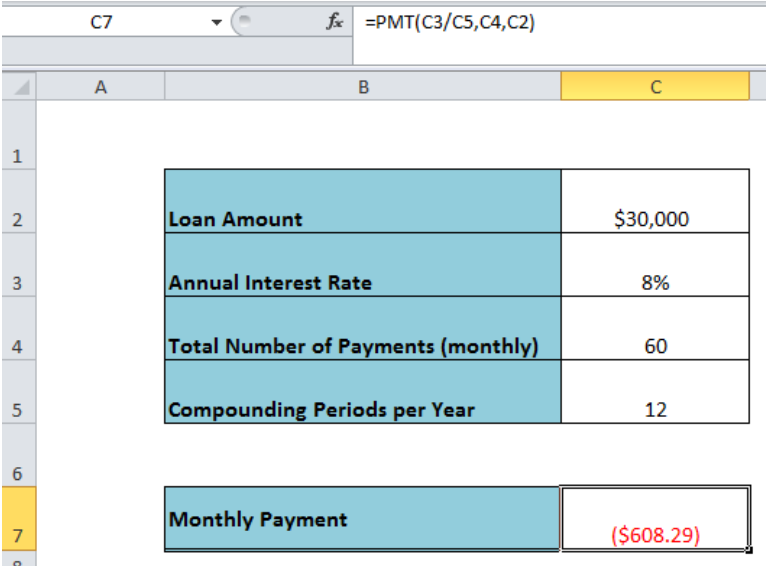Get instant live expert help with Excel or Google Sheets“My Excelchat expert helped me in less than 20 minutes, saving me what would have been 5 hours of work!”

#### Post your problem and you’ll get Expert help in seconds.

Your message must be at least 40 characters
Our professional Expert are available now. Your privacy is guaranteed.

# How to Calculate Payment For a Loan in Excel

While taking a loan from a bank or a financial institution, our primary concern is how much we have to make periodic payment (monthly, quarterly, annually) to pay back that loan on a given interest rate and in the total number of payments. Using the Excel PMT function we calculate payment for a loan based on a constant interest rate and constant payments.Figure 1. Calculating Payment For a Loan in Excel

## Formula Syntax

The syntax for the formula to calculate payment for a loan in Excel is;

`=PMT(annual rate/compounding periods, total payments, loan amount)`

OR

`=PMT(rate, nper, pv, [fv], [type])`

Where,

• Rate (required argument): A constant interest rate
• Nper (required argument): The Total number of payments or periods
• PV (required argument): Present value or the loan amount

There are two optional arguments;

• FV (optional argument): Future value of loan amount after all payments are made. Use \$0 if not known or omit this argument.
• Type (optional argument): To specify whether the payment is due at the beginning or end of the period, like at the beginning or end of the month. Use “1” for the beginning of the period and “0” for the end of the period. The default is “0Figure 2. The Syntax of the Formula

## Example

Assume we need to borrow \$30,000 at 8% annual interest rate for 5 years on monthly payment terms. We can calculate the monthly payment for this loan amount using the following formula assuming the monthly payment is due at the end of the month;

`=PMT(C3/C5,C4,C2)`Figure 3. Using the PMT Function to Calculate Payment For a Loan

As the constant interest rate is annual, so we have to convert it into monthly interest rate by dividing it to 12 (compounding periods per year). The loan period is 5 years and payments for the loan are monthly, therefore the total number of payments would be 60 (12*5). The PMT function returns payment for the loan as a negative value to represent the cash outflow, such as;Figure 4. Final Preview of Formula to Calculate Payment For a Loan

## Instant Connection to an Expert through our Excelchat Service:

Most of the time, the problem you will need to solve will be more complex than a simple application of a formula or function. If you want to save hours of research and frustration, try our live Excelchat service! Our Excel Experts are available 24/7 to answer any Excel question you may have. We guarantee a connection within 30 seconds and a customized solution within 20 minutes.

### Did this post not answer your question? Get a solution from connecting with the expert.Another blog reader asked this question today on Excelchat:
Solution exampleshow would i do this question on excel? An investment will generate Â£15,000 a year for 20 years. If you require a rate of compensation of 10% and the investment costs Â£100,000, show whether it is worth buying? If expected inflation is instead estimated to be 2% higher, show how this impacts on your recommendation.
Solved by V. W. in 60 minshow do I calculate daily interest in excel? for instance if I have \$20,000 in a bank that gives .5% interest and calculates it daily, I want to create a spreadsheet that will show what I will have at the end of a 30 day month
Solved by O. A. in 21 minsexcel if x>=Y then display Y if x<= Y then display X or 0
Solved by K. U. in 18 minsi am trying to make a box change colors based on another boxes value. the issue is I need it to change based on multiple boxes depending on which one is most recent. example, box c4 is white unless box f4 is red, then it turns red as well, however if box g4 is green then c4 is no longer red but instead turns back to white.
Solved by C. Q. in 51 minsI have Mac Excel 2016. I need to sort a column in a way which is neither ascending nor descending, and it doesn't concern days of week or months. It won't let me create my own order in custom sort.
Solved by C. A. in 60 mins## Subscribe to Excelchat.coAnother blog reader asked this question today on Excelchat: